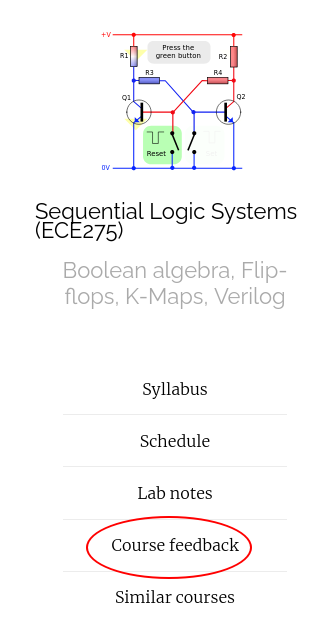## Announcments

• Homework 5 is due on Oct 15th, 9 AM before class.
• Read 3.1 to 3.33 of textbook by Wednesday.

Design a Multi-output circuit that adds two 1-bit binary numbers $$x_0$$ and $$y_0$$ and outputs a sum $$s_0$$ and carry bit $$c_1$$.
$$x_0$$$$y_0$$$$s_0$$$$c_1$$
0 0 0 0
0 1 1 0
1 0 1 0
1 1 0 1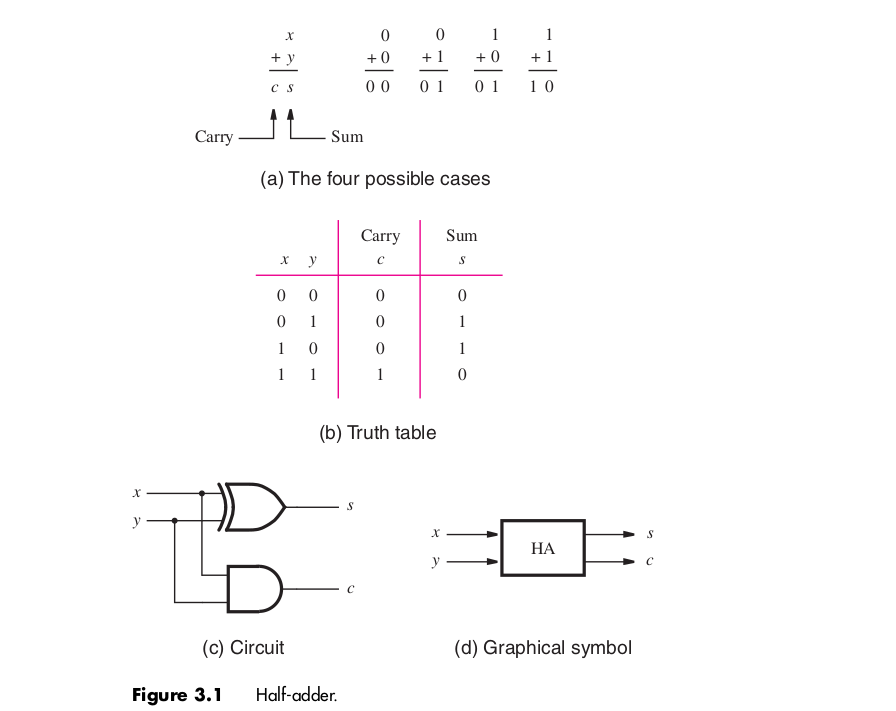Design a Multi-output circuit that adds three 1-bit binary numbers $$x_i$$, $$y_i$$ and $$c_{i}$$ outputs a sum $$s_i$$ and carry bit $$c_{i+1}$$.
$$c_i$$ $$x_i$$$$y_i$$$$s_i$$$$c_{i+1}$$
0 0 0 0 0
0 0 1 1 0
0 1 0 1 0
0 1 1 0 1
1 0 0 1 0
1 0 1 0 1
1 1 0 0 1
1 1 1 1 1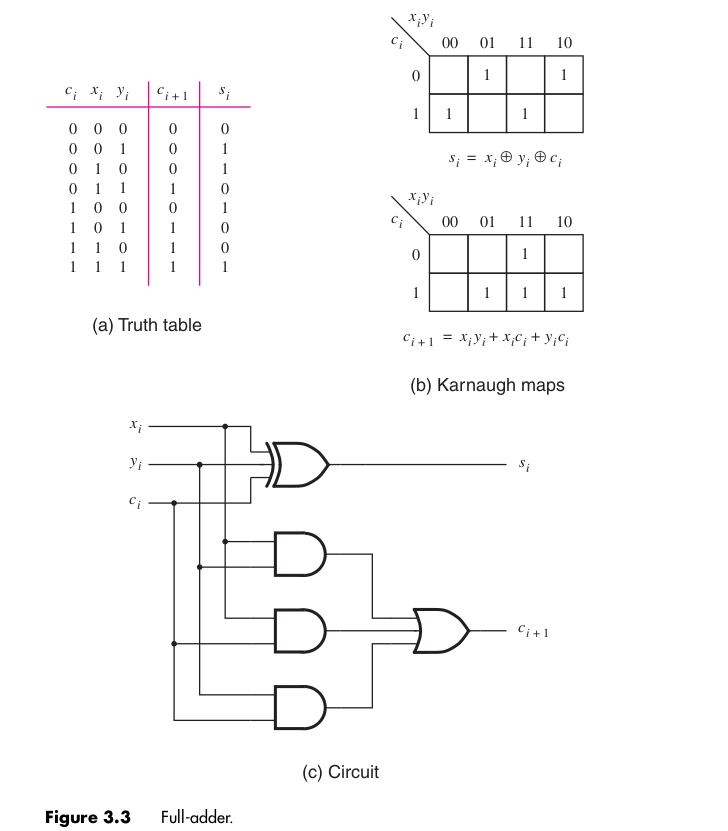Brown and Vranesic (2014)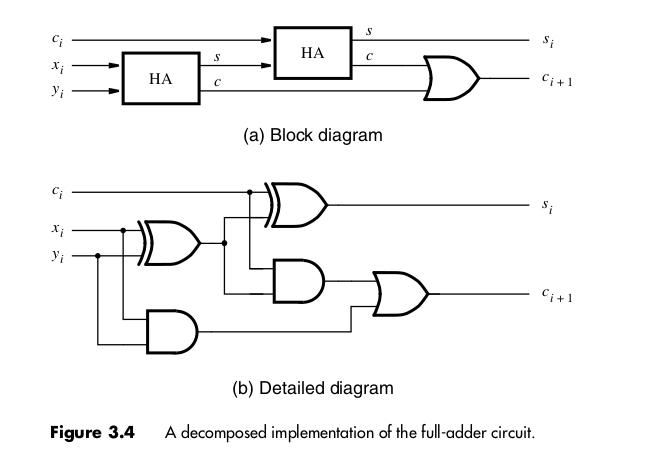Brown and Vranesic (2014)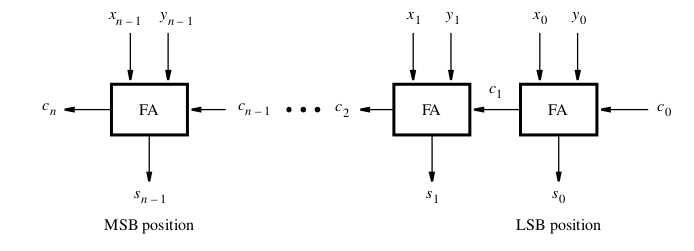Brown and Vranesic (2014)

## More circuit symbols

#### Multiplexer## Design a 4-bit Adder/Substractor unit

Using an adder and 1's complement unit.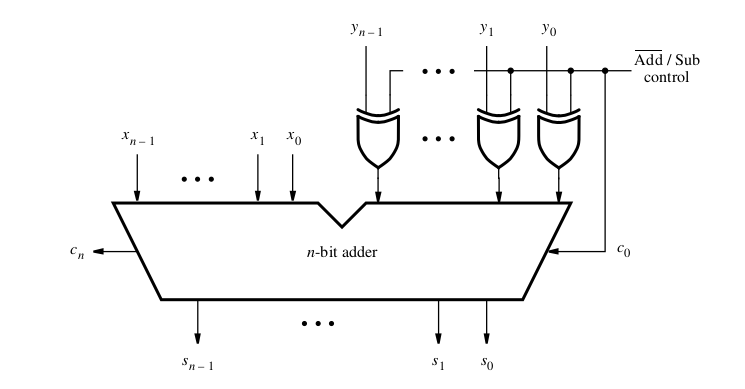https://vikasdhiman.info/ECE275-Sequential-Logic/